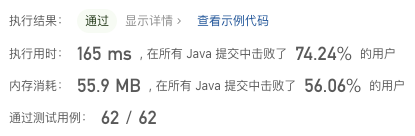#### 2208. 将数组和减半的最少操作次数

###### 来源: 每日一题 2023.07.25

``````输入：nums = [5,19,8,1]

nums 的和减小了 33 - 14.75 = 18.25 ，减小的部分超过了初始数组和的一半，18.25 >= 33/2 = 16.5 。

``````

``````输入：nums = [3,8,20]

nums 的和减小了 31 - 14.5 = 16.5 ，减小的部分超过了初始数组和的一半， 16.5 >= 31/2 = 16.5 。

``````

• `1 <= nums.length <= 105`
• `1 <= nums[i] <= 107`
``````class Solution {
public int halveArray(int[] nums) {

}
}
``````

#### 分析与题解

• 暴力方案(贪心算法 + 排序) `结果: 失败,超时 ❌❌❌`

这是我想到的第一种方式,就是我们可以每一次都是拿到数组中的最大值,然后减少一半,再添加到数组中,然后再次排序,不满足条件再次找最大值,减半,插入,排序,如此往复....

``````sum = sum / 2.0;
while(nowSum > sum) {
double lastItem = doubleNums[length - 1];
nowSum -=  lastItem - lastItem / 2.0;
doubleNums[length - 1] = lastItem / 2.0;
// 重新排序
Arrays.sort(doubleNums);
count++;
}
``````

整个解题过程如下所示.

``````class Solution {
public int halveArray(int[] nums) {
int count = 0, length = nums.length;
double sum = 0, nowSum = 0;
double[] doubleNums = new double[length];
Arrays.sort(nums);
for(int i = 0; i < length; i++) {
int item = nums[i];
sum += item;
nowSum += item;
doubleNums[i] = item;
}
sum = sum / 2.0;
while(nowSum > sum) {
double lastItem = doubleNums[length - 1];
nowSum -=  lastItem - lastItem / 2.0;
doubleNums[length - 1] = lastItem / 2.0;
Arrays.sort(doubleNums);
count++;
}
return count;
}
}
``````

复杂度分析:

• 时间复杂度: O(n㏒n)
• 空间复杂度: O(n), doubleNums长度与数组长度成正相关

• 贪心算法 + 优先队列

这种官方的解题思路和我上面的思路是差不多的,但是它利用了 `优先队列` 这个API, 先前没有怎么了解过, 今天正确的知道了它的使用方式了.

`优先队列` 内部是利用 `堆` 的实现方式,可以实现最小堆和最大堆的形式,也就是内部可进行排序......

• 那么我们需要做的工作就简单了, 直接把元素添加到 `优先队列` 中, 然后让队列自己排序.

``````PriorityQueue<Double> queue = new PriorityQueue<Double>((a, b)-> b.compareTo(a));
for(int item : nums) {
queue.offer((double)item);
sum += item;
nowSum += item;
}
``````
• 然后就是每一次取出堆的最顶部元素, 减半, 判断是否满足减半题意,不满足, 减半入堆, 进行下一次循环, 如此往复.

``````sum = sum/2.0;
while(nowSum > sum) {
double topItem = queue.poll();
nowSum -= topItem/2.0;
queue.offer(topItem/2.0);
count++;
}
``````

整个解题过程如下所示.

``````class Solution {
public int halveArray(int[] nums) {
int count = 0;
double sum = 0, nowSum = 0;
PriorityQueue<Double> queue = new PriorityQueue<Double>((a, b)-> b.compareTo(a));
for(int item : nums) {
queue.offer((double)item);
sum += item;
nowSum += item;
}
sum = sum/2.0;
while(nowSum > sum) {
double topItem = queue.poll();
nowSum -= topItem/2.0;
queue.offer(topItem/2.0);
count++;
}
return count;
}
}
``````

复杂度分析:

• 时间复杂度: O(n㏒n)
• 空间复杂度: O(n), 队列长度与数组长度成正相关...

结果如下所示.IT界无底坑洞栋主 欢迎加Q骚扰:676758285